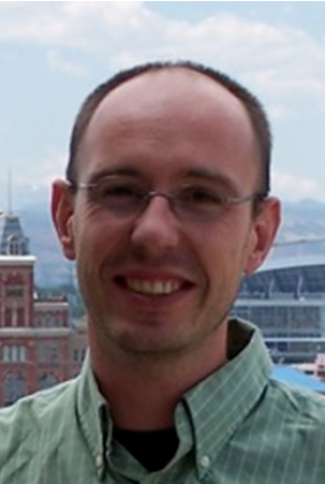# Julien LangouProfessor

Student Commons Building 4014

Expertise Areas:
Computational Mathematics

Ph.D., Applied Mathematics, INSA Toulouse, 2003
M.S., Applied Mathematics, (diplôme d'étude approfondie,) Université Paul Sabatier, Toulouse, 1999
M.S., Aerospace Engineering, (diplôme d'ingénieur,) Supaéro, Toulouse, 1999

The area of my research is Numerical Linear Algebra which is a key component of most simulation software pertaining the fact that all computational problems operate on matrices and vectors. Indeed, during a numerical simulation (weather forecasting, engineering problems, financial markets, etc.) a significant amount of time is spent in numerical linear algebra operations (say 80%). My research aims at developing better algorithms for these operations which is crucial for the scientific computing community.

There are three main components to my research: algorithms, theory, and computer programs. My vision is that all three are equally represented and emphasized. Together with my students, we are designing new algorithms which are better than previous ones in various ways: they lead to more accurate solutions, algorithms are more parallelizable, solutions are obtained faster, etc. The second aspect of my research consists of developing an understanding of the problems to be solved and of the algorithms. We develop condition number estimates and optimality bounds of the computational cost for the problems through error analysis of the algorithms and computational cost models for the algorithms. The third part of my research consists of implementing these algorithms and the experimentation thereof. While a large component of this amounts to being software engineering, there are two benefits for spending the time in implementing our algorithms in reliable and robust software; foremost this enables us to confirm the previously developed theory and secondly the advantage is that once the implementation of an algorithm is done, the software program can then be distributed to the scientific community. These three parts of my research complement themselves nicely. Understanding the theory leads to insights on how to improve algorithms. Computer program experimentation helps us to detect weaknesses in an algorithm and therefore enables us to find in which direction the algorithm design needs to proceed.

MATH 1401: Calculus I
MATH 3191: Applied Linear Algebra
MATH 3195: Differential Equations & Linear Algebra
MATH 4310: Introduction to Real Analysis I
MATH 4320: Introduction to Real Analysis II
MATH 4650: Numerical Analysis I
MATH 4733: Partial Differential Equations
MATH 4810: Probability
MATH 5070: Applied Analysis
MATH 5660: Numerical Analysis I
MATH 5718: Applied Linear Algebra
MATH 5733: Partial Differential Equations
MATH 7664: Iterative Methods in Numerical Linear Algebra
MATH 7665: Numerical Linear Algebra